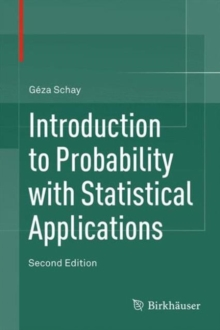Supporting your high street Find out how »
• My AccountIntroduction to Probability with Statistical Applications Hardback

Description

Now in its second edition, this textbook serves as an introduction to probability and statistics for non-mathematics majors who do not need the exhaustive detail and mathematical depth provided in more comprehensive treatments of the subject.

The presentation covers the mathematical laws of random phenomena, including discrete and continuous random variables, expectation and variance, and common probability distributions such as the binomial, Poisson, and normal distributions.

More classical examples such as Montmort's problem, the ballot problem, and Bertrand's paradox are now included, along with applications such as the Maxwell-Boltzmann and Bose-Einstein distributions in physics. Key features in new edition:* 35 new exercises* Expanded section on the algebra of sets * Expanded chapters on probabilities to include more classical examples* New section on regression* Online instructors' manual containing solutions to all exercises

Each new concept is clearly explained and is followed by many detailed examples. ... numerous examples of calculations are given and proofs are well-detailed." (Sophie Lemaire, Mathematical Reviews, Issue 2008 m)

Information

• Format: Hardback
• Pages: 385 pages, 49 Illustrations, black and white; XII, 385 p. 49 illus.
• Publisher: Birkhauser
• Publication Date:
• Category: Integral calculus & equations
• ISBN: 9783319306186

Other Formats

£64.99

£53.75

on all orders

Pick up orders

from local bookshops

£40.99

£34.65

£40.99

£34.84

£55.00

£46.75

£64.99

£55.24